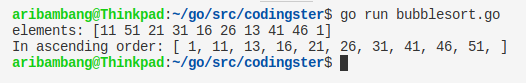-->

## Social Items

Bubble Sort Algorithm in Go (Golang) - This code implements the bubble sort algorithm to sort numbers in ascending order. Bubble Sort is less efficient as its average and worst case complexity is high, there are many other fast sorting algorithms like quick-sort, heap-sort, etc.

### Source Code :

``````package main

import "fmt"

func bubbleSort(arr []int) {
for {
sorted := true
for i := 0; i < len(arr)-1; i++ {
if arr[i] > arr[i+1] {
tmp := arr[i]
arr[i] = arr[i+1]
arr[i+1] = tmp
sorted = false
}
}
if sorted == true {
break
}
}
}

func main() {
arr := []int{11, 51, 21, 31, 16, 26, 13, 41, 46, 1}
fmt.Print("elements: ", arr, "\n", "In ascending order: [ ")
bubbleSort(arr)
for i := 0; i < len(arr); i++ {
fmt.Print(arr[i], ", ")
}
fmt.Print("]\n")
}
``````

### Compile & Run :

Here's how to compile source code manually:
``````
\$ go build bubblesort.go
\$ ./bubblesort
``````
Run without compile:
``````
\$ go run bubblesort.go
``````

### Output Of Program :Result program bubble sort in Golang## Bubble Sort Algorithm in Go (Golang)

Bubble Sort Algorithm in Go (Golang) - This code implements the bubble sort algorithm to sort numbers in ascending order. Bubble Sort is less efficient as its average and worst case complexity is high, there are many other fast sorting algorithms like quick-sort, heap-sort, etc.

### Source Code :

``````package main

import "fmt"

func bubbleSort(arr []int) {
for {
sorted := true
for i := 0; i < len(arr)-1; i++ {
if arr[i] > arr[i+1] {
tmp := arr[i]
arr[i] = arr[i+1]
arr[i+1] = tmp
sorted = false
}
}
if sorted == true {
break
}
}
}

func main() {
arr := []int{11, 51, 21, 31, 16, 26, 13, 41, 46, 1}
fmt.Print("elements: ", arr, "\n", "In ascending order: [ ")
bubbleSort(arr)
for i := 0; i < len(arr); i++ {
fmt.Print(arr[i], ", ")
}
fmt.Print("]\n")
}
``````

### Compile & Run :

Here's how to compile source code manually:
``````
\$ go build bubblesort.go
\$ ./bubblesort
``````
Run without compile:
``````
\$ go run bubblesort.go
``````

### Output Of Program :Result program bubble sort in Golang